Minimizing Parameters for the Geodesic's Quadratic Approximation

Does the total length of the quadratic approximation have a minimum with respect to its remaining parameter?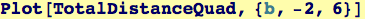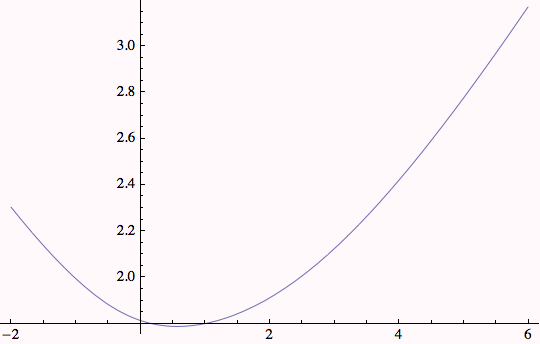OK, the total distance is a minimum somewhere on the interval 0 < b < 1.  Solving for the value of b at the minimum: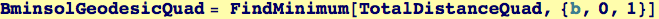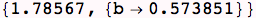Now we can use the minimizing b to find the best quadratic approximation to the geodesic.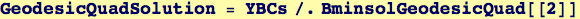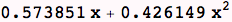This is the shape of the path, it doesn't differ very much from a straight line.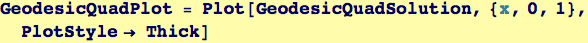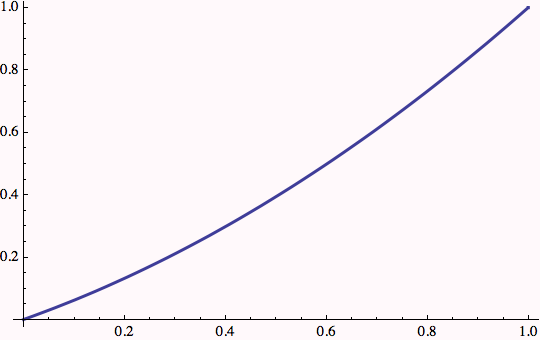Created by Wolfram Mathematica 6.0  (26 November 2007)